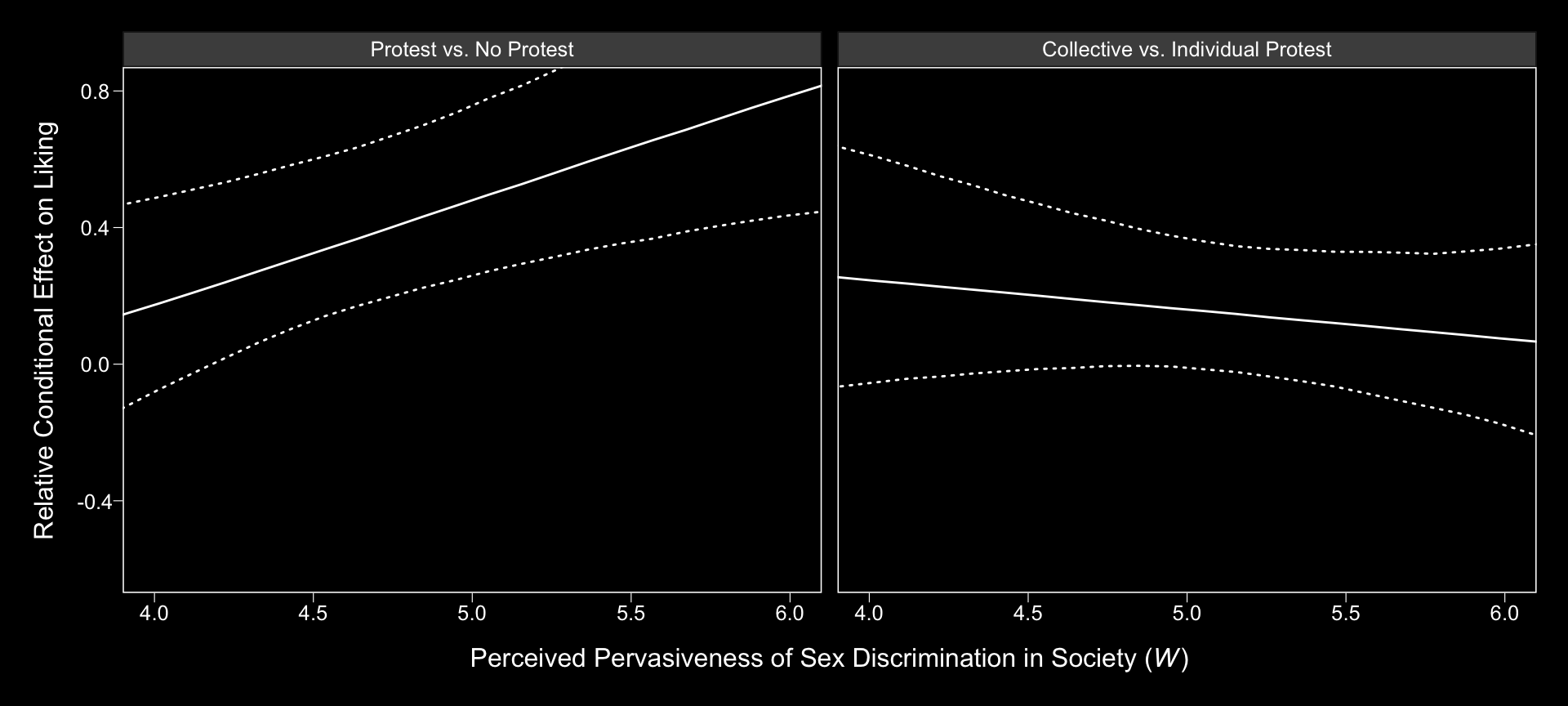## 13.3 Relative conditional indirect effects

Before we use Hayes’s formulas at the top of pate 488 to re-express the posterior in terms of the relative conditional indirect effects, we might want to clarify which of the post columns correspond to the relevant parameters.

• $$a_{1}$$ = b_respappr_D1
• $$a_{2}$$ = b_respappr_D2
• $$a_{4}$$ = b_respappr_D1:sexism
• $$a_{5}$$ = b_respappr_D2:sexism
• $$b$$ = b_liking_respappr

To get our posterior transformations, we’ll use the purrr::map() approach, which first requires we define two custom functions.

# (a1 + a4W)*b
D1_function <- function(w){
(post$b_respappr_D1 + post$b_respappr_D1:sexism*w)*post$b_liking_respappr } # (a2 + a5W)*b D2_function <- function(w){ (post$b_respappr_D2 + post$b_respappr_D2:sexism*w)*post$b_liking_respappr
}

Next, we’ll make a 30-row tibble with each row containing a value for sexism, ranging from 3.5 to 6.5. We’ll then apply our two custom functions to the sexism values and wrangle the results a bit to make them useful for our purposes.

rcie_tibble <-
tibble(sexism = seq(from = 3.5, to = 6.5, length.out = 30)) %>%
group_by(sexism) %>%
mutate(Protest vs. No Protest            = map(sexism, D1_function),
Collective vs. Individual Protest = map(sexism, D2_function)) %>%
unnest() %>%
ungroup() %>%
mutate(iter = rep(1:4000, times = 30)) %>%
gather(indirect effect, value, -sexism, -iter) %>%
mutate(indirect effect = factor(indirect effect, levels = c("Protest vs. No Protest", "Collective vs. Individual Protest")))

head(rcie_tibble)
## # A tibble: 6 x 4
##   sexism  iter indirect effect         value
##    <dbl> <int> <fct>                     <dbl>
## 1    3.5     1 Protest vs. No Protest  0.160
## 2    3.5     2 Protest vs. No Protest -0.0305
## 3    3.5     3 Protest vs. No Protest -0.174
## 4    3.5     4 Protest vs. No Protest -0.143
## 5    3.5     5 Protest vs. No Protest -0.118
## 6    3.5     6 Protest vs. No Protest  0.00955

Here is our variant of Figure 13.4, with respect to the relative conditional indirect effects.

rcie_tibble %>%
group_by(indirect effect, sexism) %>%
summarize(median = median(value),
ll = quantile(value, probs = .025),
ul = quantile(value, probs = .975)) %>%

ggplot(aes(x = sexism, group = indirect effect)) +
geom_ribbon(aes(ymin = ll, ymax = ul),
color = "white", fill = "transparent", linetype = 3) +
geom_line(aes(y = median),
color = "white") +
coord_cartesian(xlim = 4:6,
ylim = c(-.6, .8)) +
labs(x = expression(paste("Perceived Pervasiveness of Sex Discrimination in Society (", italic(W), ")")),
y = "Relative Conditional Effect on Liking") +
theme_black() +
theme(panel.grid = element_blank(),
legend.position = "none") +
facet_grid(~ indirect effect)Here are the indirect effect summaries relevant to the contents of Table 13.2.

rcie_tibble_pick_a_point <-
# notice how we've restricted ourselves to only 3 values for sexism
tibble(sexism = c(4.25, 5.125, 5.896)) %>%
group_by(sexism) %>%
mutate(Protest vs. No Protest = map(sexism, D1_function),
Collective vs. Individual Protest = map(sexism, D2_function)) %>%
unnest() %>%
ungroup() %>%
# because we only have 3 sexism values, we only need to repeat times = 3
mutate(iter = rep(1:4000, times = 3)) %>%
gather(indirect effect, value, -sexism, -iter) %>%
mutate(indirect effect = factor(indirect effect, levels = c("Protest vs. No Protest", "Collective vs. Individual Protest")))

rcie_tibble_pick_a_point %>%
group_by(sexism, indirect effect) %>%
summarize(median = median(value),
ll = quantile(value, probs = .025),
ul = quantile(value, probs = .975)) %>%
mutate_if(is.double, round, digits = 3)
## # A tibble: 6 x 5
## # Groups:   sexism 
##   sexism indirect effect                 median     ll    ul
##    <dbl> <fct>                              <dbl>  <dbl> <dbl>
## 1   4.25 Protest vs. No Protest             0.248  0.03  0.538
## 2   4.25 Collective vs. Individual Protest  0.225 -0.033 0.545
## 3   5.12 Protest vs. No Protest             0.518  0.288 0.807
## 4   5.12 Collective vs. Individual Protest  0.15  -0.021 0.347
## 5   5.90 Protest vs. No Protest             0.755  0.423 1.16
## 6   5.90 Collective vs. Individual Protest  0.083 -0.152 0.331